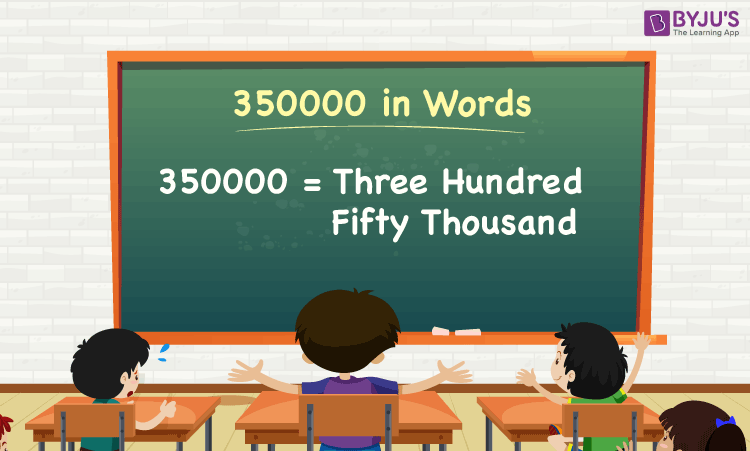# 350000 in Words

350000 in words is Three Hundred Fifty Thousand. For example, if you spent Rs. 350000 in 9 months, you can write, “I have spent Rs. Three Hundred Fifty Thousand in nine months”. Basically, the number name of any number can be written using the ones, tens, hundreds, and thousands place of a number. Thus, the place value chart is useful for writing the number 350000 in words.

 350000 in Words: Three Hundred Fifty Thousand

In this article, let us learn how to write 350000 in words, and solved examples in detail.

## How to Write 350000 in Words?As discussed earlier, to write the number name of 350000, first, we have to determine the place value of each digit of the given number 350000.

That is, 1’s place of 350000 is 0

10’s place of 350000 is 0.

100’s place of 350000 is 0.

1000’s place of 350000 is 350.

The following chart represents the place value of the number 350000:

 Thousands Hundreds Tens Ones 350 0 0 0

Therefore, the number 350000 in words is Three Hundred Fifty Thousand.

### Examples

Example 1:

Determine the value of 500000 – 150000, and express the value in words.

Solution:

Given expression: 500000 – 150000

⇒ 500000 – 150000 = 350000

So, the value of 500000 – 150000 is 350000.

Hence, 350000 in words is Three Hundred Fifty Thousand.

Example 2:

Describe the value of three hundred fifty thousand plus ten thousand in words.

Solution:

Three Hundred Fifty Thousand = 350000

Ten thousand = 10000

Three hundred fifty thousand plus ten thousand = 350000 + 10000 = 360000

Hence, the value of three hundred fifty thousand plus ten thousand is three hundred sixty thousand in words.

Stay tuned to BYJU’S – The Learning App, and download the app to learn all Maths concepts quickly.

## Frequently Asked Questions on 350000 in Words

Q1

### Write 350000 in words.

350000 in words is Three Hundred Fifty Thousand.

Q2

### Express the value 350500 – 500 in words.

Simplifying 350500 – 500, we get 350000. Hence, 350000 in words is three hundred fifty thousand.

Q3

### How to write three hundred fifty thousand in numbers?

Three hundred fifty thousand in numbers is 350000.

Q4

### Is the number 350000 an odd number?

No, the number 350000 is not an odd number.

Q5

### Is 350000 a perfect square number?

No, 350000 is not a perfect square number.